# Absolutely absolute

Algebra Level 3

Below is the graph of $y=f(x)$: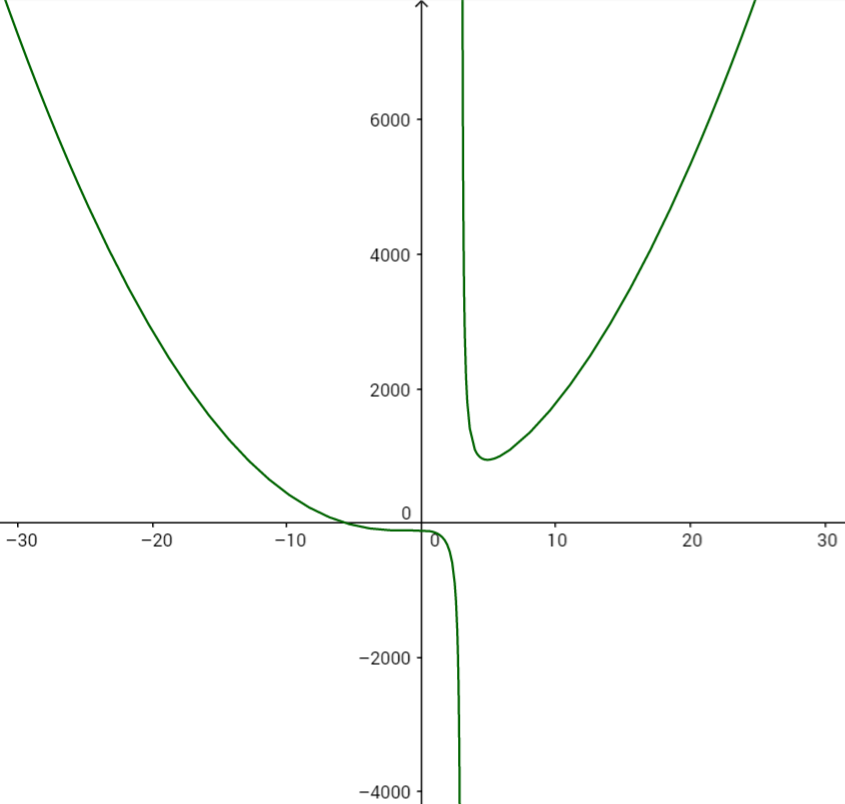Match the following graphs with the correct equations:

• $y_1=f(|x|)$
• $y_2=|f(x)|$
• $y_3=|f(-x)|$
• $y_4=|f(|x|)|$

Graph A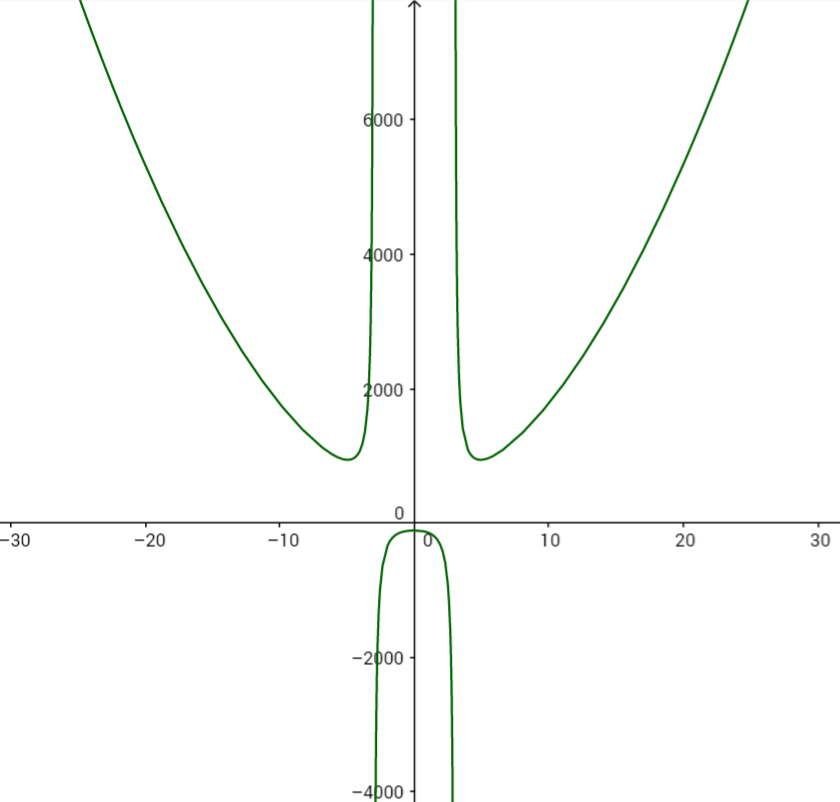Graph B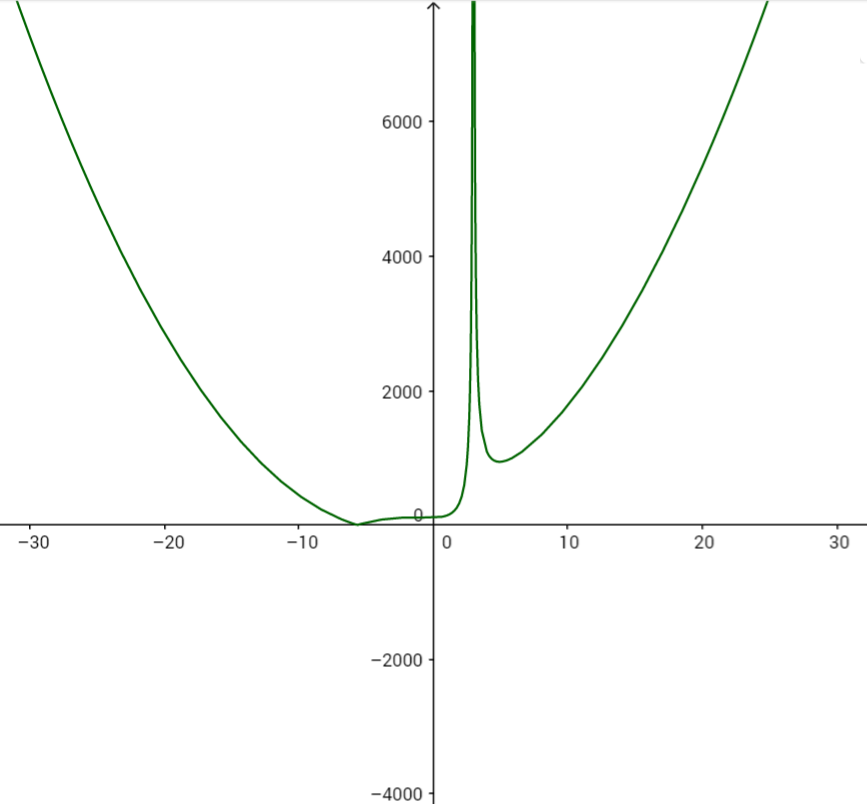Graph C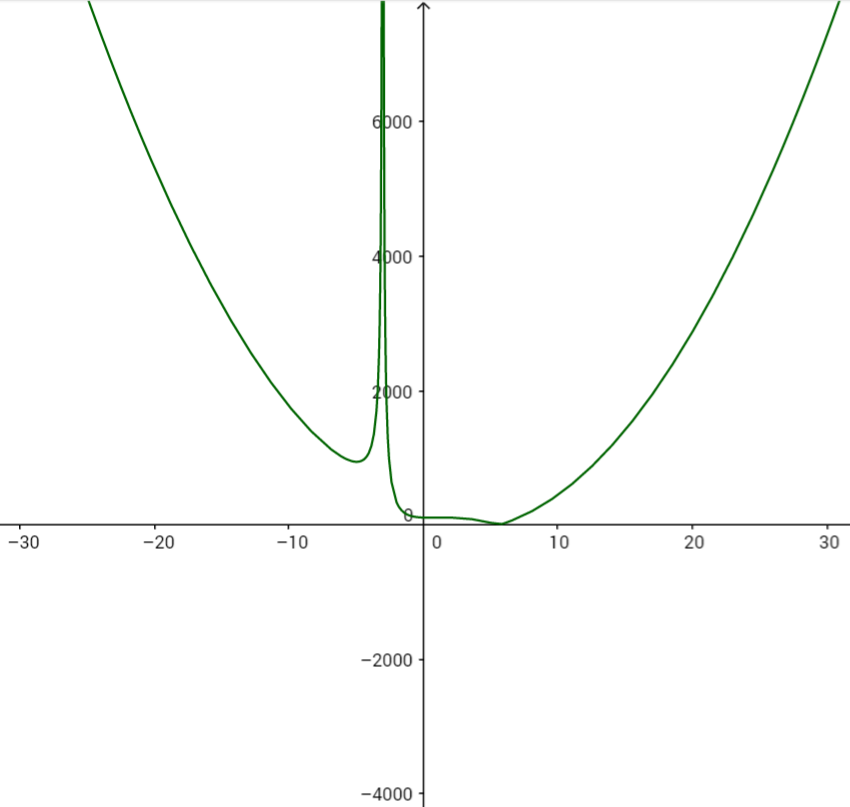Graph D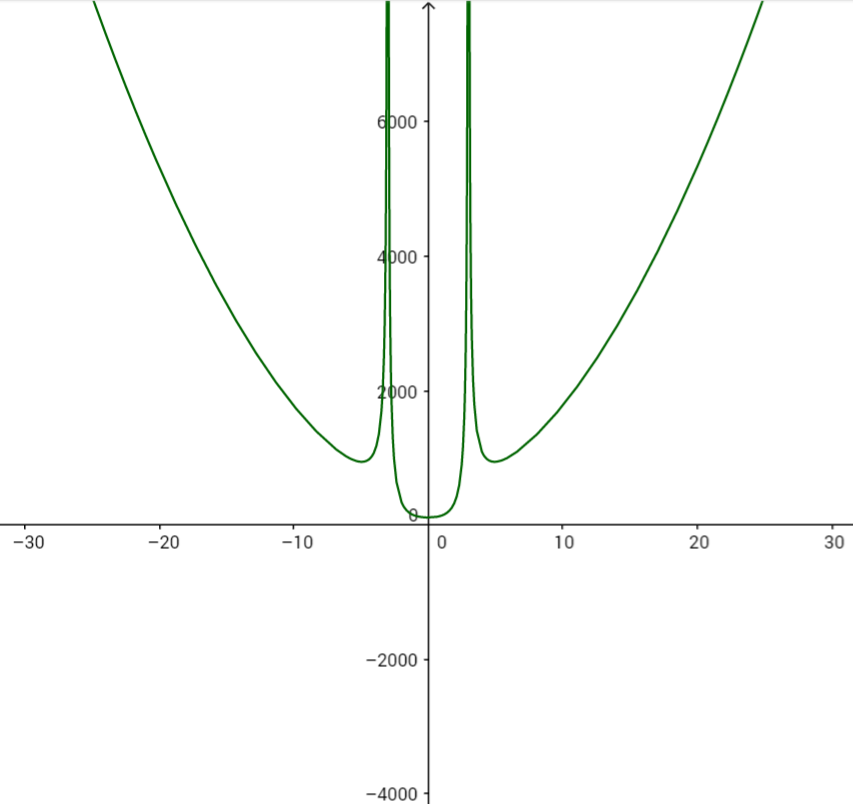×